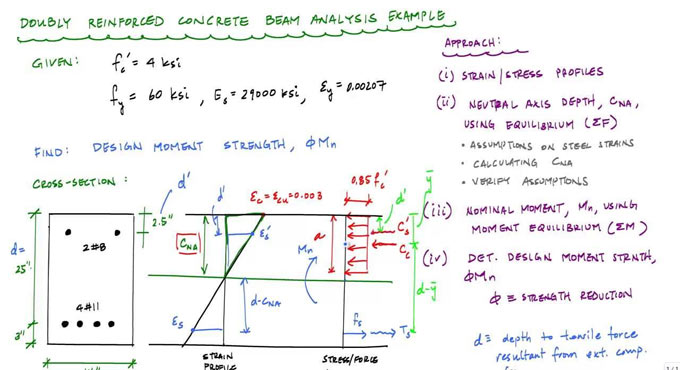# A wide array of reinforced concrete design examplesThis construction article is based on various reinforced concrete design examples. You will be familiar with flexural analysis of beam.

Given below, various examples and their solutions :-
Make proper calculation for the following reinforced concrete sections :-

Example 1 : The balanced steel reinforcement: The maximum steel reinforcement area for a tension-controlled and transition section per ACI code 318-11.

The location of the neutral axis and the depth of the equivalent compressive Whitney stress block for the tension-controlled section in B.

Here, the compressive strength is given as f'c = 4 ksi and yield strength is given as Fy = 60 ksi

ACI code 318-11 is followed

Example 2 : Examine the adequacy of a rectangular tension controlled section on the basis of dead and live loads.

A 10 ft long cantilever beam contains a rectangular section and reinforcement. The beam bears a dead load of 2 k/ft (along with self weight) and a live load of 1 k/ft.

The compressive strength is provided as f'c = 4 ksi and yield strength is fy = 60 ksi, verify if the beam has sufficient strength to bear the provided loads with ACI Code 318-11.

Example 3 : Work out the design moment strength and the location of the neutral axis of a rectangular section containing two rows of tension reinforcement.

b (width) is given as 13 inches
d is given as 23.5
h (through depth of the section) is given as 27 inches
dt (distance from the extreme compression fibre to the location of the extreme tension reinforcement) is given as 24.5

f'c (the compressive strength) is given as 4 ksi
fy (the yield strength) is given as 60 ksi

Example 4 : Work out the design moment strength and the position of the neutral axis of a rectangular section with compression reinforcement that yields.

The following properties are included in the rectangular section :-
Width = b = 12"
Effective depth = d = 22.5"
Tension reinforcement = (6) no. 9 bars
Compression reinforcement = (2) no. 6 bars

Compute the design strength of the beam if f'c = 4 ksi and fy = 50 ksi with ACI Code 318-11.

To get the solutions of the above-mentioned problems, go through the following link.